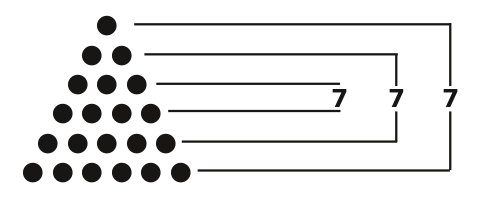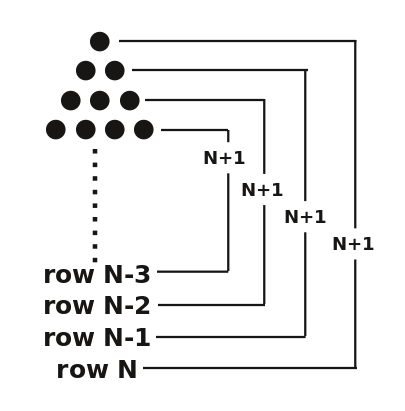Use this Python script to simulate Babbage's Difference Engine

Nineteenth-century mathematician Charles Babbage's serial calculation machine meets its match with Python code.Image credits :

Subscribe now

Get the highlights in your inbox every week.

Charles Babbage (1791–1871) was an avid mathematician with very wide interests. He is well-known for envisioning the idea of computers and single-handedly developed what he called a Difference Engine to make serial calculations. It was a mechanical machine with a series of axles and gears to make calculations, with the output being a printed table. I recently began reading his 1864 book, Passages from the Life of a Philosopher, where he explains how the Difference Engines came to be.

One of the problems his Engine was designed to solve relates to the idea of children playing with marbles and arranging them in a progressive pyramidal shape, with one marble in the top row, two in the second, three in the third, and so on. For small pyramids, you can simply count the marbles to find how many there are. But Babbage wanted to create an automatic list or table with one column showing the number of rows and another column showing the total number of marbles.

babbage_marblepyramid.png(Greg Pittman, CC BY-SA 4.0)

The differences he talks about are first, all the successive differences in the number of rows (equal to one in this example), and second, the difference between the number of marbles added from one row to the next (also one). As I understand it, the Difference Engine successively adds the number of marbles for each new row to the previous total, creating the table in the process.

And so I began playing with marbles in my head, so to speak. I found myself awake in bed at 5am mentally fiddling with and counting marbles. One thing that struck me was that for six rows, the total was 21, and for seven, it was 28—both evenly divisible by seven. For eight or nine rows, the total was divisible by nine. As I went higher up the number of rows, this pattern recurred. All of this was in my head (meanwhile, I was wishing it would stop so that I could get back to sleep), so it wasn't very systematized.

When I woke up, I decided to try to figure out why this was and whether I could predict which numbers would show this phenomenon. The answer surprised me: It turns out that every odd number of rows will have a number of marbles evenly divisible by that number.

But how could I prove this? Here is an example. For the pyramid with seven rows, look first at the pyramid with six rows, with six marbles across the bottom. Create some pairs of rows: the first and the sixth, the second and the fifth, the third and the fourth. For each of these three pairs, the sum is seven; thus, the total of the three sets of pairs is 21, and if you add seven for the next row, it would also remain evenly divisible by seven.

babbage_pyramidpairs.png(Greg Pittman, CC BY-SA 4.0)

You can keep doing this with specific examples, but the goal is to understand this phenomenon generally. Imagine some random even number of rows, N. Create your pairs, then add 1 to N, 2 to N-1, 3 to N-2, and so on. In each case, the sum will be N+1. The number of these pairs will be N/2, so the total number of marbles will be N/2*(N+1), a number evenly divisible by N+1. Adding N+1 marbles for the next row is also evenly divisible by N+1.

babbage_pyramidnpairs.png(Greg Pittman, CC BY-SA 4.0)

For example, you can say that a pyramid with 128,948 rows will have a total of 8,313,857,826 marbles; a pyramid with 128,949 rows will have 8,313,986,775, and both these totals will be evenly divisible by 128,949.

I don't know if Babbage considered this, but chances are, even if he did, he would say this just represents mathematical problems of multiplying and dividing six-digit numbers, and he wanted a machine to do that kind of thing. He did envision and begin work on an Analytical Engine, which perhaps could have done this sort of operation.

Nowadays, this is trivial work for computers, so here's a Python script to count your marbles before you lose them—in just 4 lines of code.

#!/usr/bin/env python
# babbage.py
"""
Using Charles Babbage's conception of a marble-counting
operation for a regular pyramid of marbles,starting with
one at the top with each successive row having one more
marble than the row above it.

Returns total number of marbles in a pyramid of any size.
"""

MarbRows = input("Enter the number of rows of marbles:  ")
MarbRows = int(MarbRows)

MarbNum = int((MarbRows)*(MarbRows+1)/2)
print ("The number of marbles is "+ str(MarbNum))

And this is how Babbage's Difference Engine meets Python.